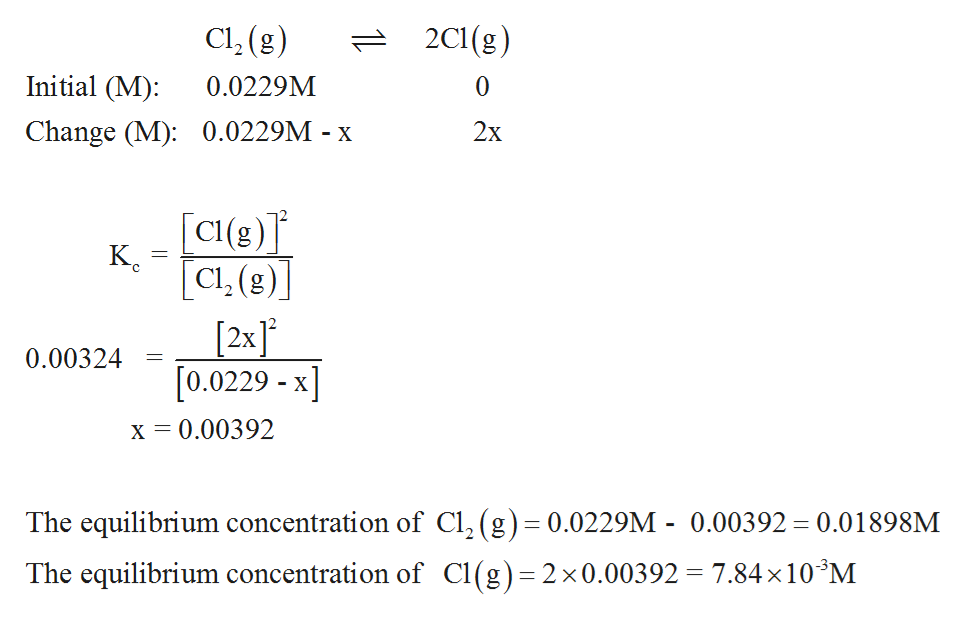# At a certain temperature, the equilibrium constant, Kc , is 0.00324 for the reactionCl2(g)−⇀↽−2Cl(g)A. If 3.24 g Cl2 is placed in a 2.00 L flask at this temperature, what are the equilibrium concentrations of Cl2 and Cl ?B. Following the establishment of equilibrium in part A, the volume of the flask is suddenly increased to 5.00 L while the temperature is held constant. What are the new equilibrium concentrations of Cl2 and Cl ?C. Following the establishment of equilibrium in part A, the volume of the flask is instead suddenly decreased to 1.00 L while the temperature is held constant. What are the new equilibrium concentrations of Cl2 and Cl ?

Question
2 views

At a certain temperature, the equilibrium constant, Kc , is 0.00324 for the reaction

Cl2(g)−⇀↽−2Cl(g)

A. If 3.24 g Cl2 is placed in a 2.00 L flask at this temperature, what are the equilibrium concentrations of Cl2 and Cl ?

B. Following the establishment of equilibrium in part A, the volume of the flask is suddenly increased to 5.00 L while the temperature is held constant. What are the new equilibrium concentrations of Cl2 and Cl ?

C. Following the establishment of equilibrium in part A, the volume of the flask is instead suddenly decreased to 1.00 L while the temperature is held constant. What are the new equilibrium concentrations of Cl2 and Cl ?

check_circle

Step 1

A)

The Kc expression for given reaction is as follows,

Step 2

The moles of Cl2 present is determined by using given mass and its molar mass.

Step 3

The initial concentration of Cl2 is 0.0457 mol/2L = 0.0229M. Observing the balanced equation shows that one mole of Cl2 given 2 moles of...help_outlineImage TranscriptioncloseCl, (g) 2C1(g) Initial (M): 0.0229M Change (M): 0.0229M - x 2x [C(g)]* K. [Cl. (g)] [2x]° [0.0229 - x] 0.00324 х 3 0.00392 The equilibrium concentration of Cl, (g)= 0.0229M - 0.00392 = 0.01898M The equilibrium concentration of Cl(g)=2x0.00392 = 7.84×10*M fullscreen

### Want to see the full answer?

See Solution

#### Want to see this answer and more?

Solutions are written by subject experts who are available 24/7. Questions are typically answered within 1 hour.*

See Solution
*Response times may vary by subject and question.
Tagged in

### Physical Chemistry